# Dinosaurs

More than 30 and less than 60 dinosaurs have met at the pond. A quarter of them bathed and 1/7 saws and the rest gripped. How many were at the pond? How many were there?

Correct result:

n =  56
j =  8

#### Solution:

$j=n\mathrm{/}7=56\mathrm{/}7=8$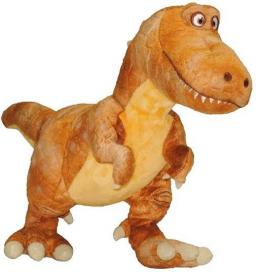We would be very happy if you find an error in the example, spelling mistakes, or inaccuracies, and please send it to us. We thank you!Tips to related online calculators
Do you want to calculate least common multiple two or more numbers?

## Next similar math problems:

• Lesson exercising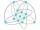The lesson of physical education, pupils are first divided into three groups so that each has the same number. The they redistributed, but into six groups. And again, it was the same number of children in each group. Finally they divided into nine equal g
• School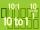Headteacher think whether the distribution of pupils in race in groups of 4,5,6,9 or 10. How many pupils must have at least school at possible options?
• Balls groups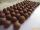Karel pulled the balls out of his pocket and divide it into the groups. He could divide them in four, six or seven, and no ball ever left. How little could be a ball?
• Lcm of three numbersWhat is the Lcm of 120 15 and 5
• Counting numberWhat is the smallest counting number divisible by 2,5,7,8 and 15?
• The smallest numberWhat is the smallest number that can be divided by both 5 and 7
• Lcm simpleFind least common multiple of this two numbers: 140 175.
• LCMWhat is the least common multiple of 5, 50, 14?
• The port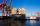Four ships Berthed in the port. Together sail from a port. The first ship will return to port every two weeks, second ship after 4 weeks, third after 8 weeks and fourth ship after 12 weeks. After how many weeks all the boats gather at the port?
• Bus linesAt 5.00 in the morning, four buses left the terminal at the same time. The first bus has an interval of 15 minutes, the second 20 minutes, the third 25 minutes, and the fourth 45 minutes. How many minutes will all four buses leave the terminal at the same
• 3 buses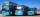At morning 5:00 am three buses started from one place. The first travel in five-minute intervals, the second at 10-minute intervals and the third at 25-minute intervals. At what hour will again be the three buses coming from the same place?
• Write decimalsWrite in the decimal system the short and advanced form of these numbers: a) four thousand seventy-nine b) five hundred and one thousand six hundred and ten c) nine million twenty-six
• ProductResult of the product of the numbers 1, 2, 3, 1, 2, 0 is:Added together and write as decimal number: LXVII + MLXIVWrite numbers written in Roman numerals as decimal.Factor the number 6600 into the product of prime numbers.Ski organizers should print the start numbers from 1 to 45. How many times will they use the number 3 when printing?